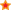for Secondary Education in the UK.Find Resources By Subjects
Information

# Catching up on Numeracy: Mental Arithmetic

Price: £25.00
Age Range: KS3

Topics include: Strategies and short cuts for multiplication and division; Number bonds; percentages and money; Calculations using weights, volume, length and time; Square numbers; Factors; Prime numbers; Fractions; Negative numbers in the context of temperature.

Quantity Format
Size: 68 Pages
ISBN: 978 1 86025 213 6
Code: CNMA
Popularity rank: 28
Average Customer Review:based on 2 reviews

# Contents List

#### LESSONS

• 7 COUNT OUT LOUD This page helps students to develop their mental agility through practice, and suggests imagining movement along a number line in regular steps as an aid to developing mental arithmetic skills.
• 9 BONDS By practising number bonds up to ten, and then extending this to those for 100 and 1000, this page aims to encourage students to recognise pairs that bond. This will then aid them in the mental addition of large numbers.
• 11 TENS AND UNITS This page encourages students to practise the addition of numbers of less than 100 in situations where they deal with sums of money of less than £1 - and to understand that such calculations are two-step mental operations.
• 13 BACKWARDS This page encourages 'mental flexibility' in counting backwards through practice, and continues the idea of imagining movement along a number line in regular steps.
• 15 DOUBLE VISION This page helps students develop their ability to double any number from 0 to 100.
• 17 BUILDING NUMBERS This page develops appreciation of place value as a fundamental aspect of number systems. Students practise using place value to simplify mental arithmetic multiplications by 10, 100 and 1000.
• 19 TABLE TALK Students are made familiar with the first 15 multiples of 3 and 7 by using a 'flash card' system.
• 21 WHERE'S THE POINT? The main activity of this page develops students' appreciation of place value and its use in simplifying calculations, involving division by 10 and 100.
• 23 TWO MINUTE CHALLENGE Students are encouraged to set themselves time targets, without sacrificing accuracy, for simple mental arithmetic exercises.
• 25 SHORT CUTS This page shows how minor adaptations of simple mental calculations can make a range of related sums easier to perform.
• 27 DOUBLE AND DOUBLE AGAIN Students are taught to see 'times by four' as a process of using a basic doubling technique twice over.
• 29 CASH QUESTIONS Students use the fact that 10% of £1 is 10p to develop the technique of mentally calculating any percentage of £1, £2 or £5, and 10% of any amount.
• 31 GO THE DISTANCE This page teaches students the relationships between millimetres, centimetres and metres, and gets them to apply these in a range of mental arithmetic calculations.
• 33 TEMPERATURE This page introduces negative numbers in the context of temperatures, and encourages students to envisage a thermometer to help them understand the differences between numbers.
• 35 BY TEN AND THEN... This page develops the skill of multiplying by multiples of 10, by presenting it as a two-stage process.
• 37 LUCRETIA'S PIZZAS This page provides a visual reminder to students of how to find equivalent fractions, and reinforces the techniques with useful exercises.
• 39 QUICK CHANGE Students practise using number bonds for 20 and 50 with the aim of encouraging immediate confidence in giving change for 20p and 50p.
• 41 SWIMMING POOLS Students will have to remember certain basic formulas as part of the SAT mental arithmetic test. This page prepares students for the calculation of the area of simple rectangles.
• 43 FACTS ABOUT FACTORS This page promotes mental arithmetic agility by helping students to understand the term 'factor'. Students practise finding factors of numbers up to 40.
• 45 MORE SHORT CUTS This page enables students to see subtraction of numbers close to 10 as a two-stage mental process.
• 47 FIGHTING FIT This page aims to reinforce students' understanding that there are 1000 grams in one kilogram.
• 49 RANCHING AND PLUMBING Through comparing square and non-square areas, students investigate the concept of square numbers, working within th range of the first 20. Aims
• 51 THE POWERS OF TWO Doubling the number 2 is used as a means of introducing index notation. This page aims to ensure that students understand that, for example, 2 to the power of 5 is 32 (not 10).
• 53 CUT IN HALF This page aims to encourage visualisation of mental arithmetic calculations involving division by 2 as a process of separation of hundreds, tens and units.
• 55 HAVE YOU THE TIME? The main activity on this page reminds students that adding minutes to a given time is not a decimal operation because there are 60 minutes in an hour.
• 57 SQUARE ROOTS Understanding the term 'square root' and investigating the first ten square numbers is the main activity on this page.
• 59 PRIME NUMBERS This page reinforces students' understanding of prime numbers.
• 61 TRIPLE HEADER Students are encouraged to develop mental arithmetic strategies for the addition of three numbers that have no more than two digits each.
• 63 COUNT DOWN A series of counting aloud exercises reminds students of place value for numbers below one and encourages their confidence with number lines.
• 65 FAIRGROUND This page introduces the idea that circumference is approximately three times the diameter, in any circle. Estimations of circumference are sought with increasing degrees of accuracy.
• 66 APPENDIX Grids for students to use for the final activity on TWO MINUTE CHALLENGE (page 23).

## Reviews

20/11/07

### Name and school withheld.

Interesting, informative and fun. My pupils like working from these sheets.